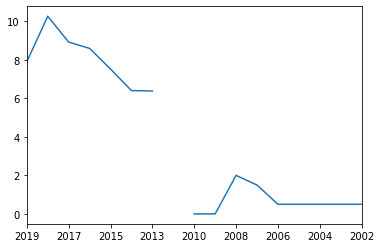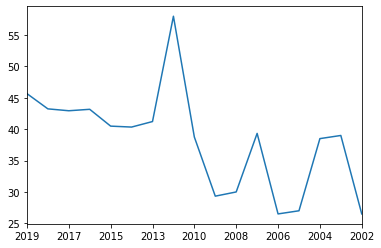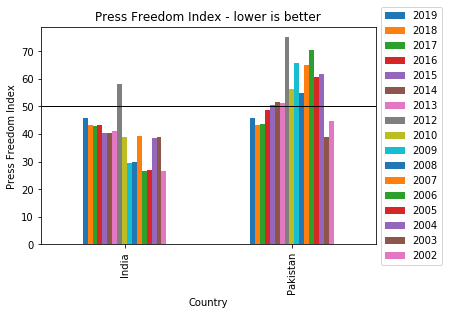# How To Analyze Wikipedia Data Tables Using Python Pandas

In this post, I will show you how to plot graphs from the Wikipedia data tables.

I have copied data 'Press Freedom Index' from following page. 'Press Freedom Index' ranks Countries every year based on the Press Freedom. Lower the number better the Press Freedom.

en.wikipedia.org/wiki/Press_Freedom_Index

Lets first import following Python packages before doing the analysis.

In :
import pandas as pd
from matplotlib.pyplot import hist
import seaborn as sns
import numpy as np


Our data is in following file 'journalism_index.csv'

In :
df = pd.read_csv('journalism_index.csv')


Let us take a peek in to our data and see how it looks.

In :
df.head(3)

Out:
Country 2019 2018 2017 2016 2015 2014 2013 2012 2010 2009 2008 2007 2006 2005 2004 2003 2002
0 NaN        [a]         
1 Norway -1 -1 -1 -3 -2 -3 -3 -1 -1 -1 -1 -1 -6 -1 -1 -1 -1
2 NaN 7.82 7.63 7.6 8.79 7.75 6.52 6.52 −10.00 0 0 1.5 0.75 2 0.5 0.5 0.5 0.5

As we see above, our data is shifted. Country and values are not aligned. We need to fix our data first.

Lets pick all the rows where Country value is NaN. We can filter out the rows using pd.isna as shown below.

In :
dfi = df[pd.isna(df.Country)==True]

In :
dfi.head(2)

Out:
Country 2019 2018 2017 2016 2015 2014 2013 2012 2010 2009 2008 2007 2006 2005 2004 2003 2002
0 NaN        [a]         
2 NaN 7.82 7.63 7.6 8.79 7.75 6.52 6.52 −10.00 0 0 1.5 0.75 2 0.5 0.5 0.5 0.5

Ok, as we see above, we got all the rows where Country is NaN. First row is not of any use. Lets drop that using dfi.drop

In :
dfi.drop([0,0],inplace=True)

In :
dfi.head(1)

Out:
Country 2019 2018 2017 2016 2015 2014 2013 2012 2010 2009 2008 2007 2006 2005 2004 2003 2002
2 NaN 7.82 7.63 7.6 8.79 7.75 6.52 6.52 −10.00 0 0 1.5 0.75 2 0.5 0.5 0.5 0.5

Let us fix the index now using reset_index()

In :
dfi = dfi.reset_index()

In :
dfi.head(1)

Out:
index Country 2019 2018 2017 2016 2015 2014 2013 2012 2010 2009 2008 2007 2006 2005 2004 2003 2002
0 2 NaN 7.82 7.63 7.6 8.79 7.75 6.52 6.52 −10.00 0 0 1.5 0.75 2 0.5 0.5 0.5 0.5
In :
dfi = dfi.drop(columns=['index'])

In :
dfi.head(2)

Out:
Country 2019 2018 2017 2016 2015 2014 2013 2012 2010 2009 2008 2007 2006 2005 2004 2003 2002
0 NaN 7.82 7.63 7.6 8.79 7.75 6.52 6.52 −10.00 0 0 1.5 0.75 2 0.5 0.5 0.5 0.5
1 NaN 7.9 10.26 8.92 8.59 7.52 6.4 6.38 −10.00 0 0 2 1.5 0.5 0.5 0.5 0.5 0.5

Ok, we need to still do following steps.

1. Replace Country Column with actual country names

Lets fix our country column first.

In :
countries = df[pd.isna(df.Country)==False]['Country'].reset_index()['Country']


countries is a Panda Series. It contains all the country names. Lets take a look in to it.

In :
countries.head()

Out:
0          Norway
1         Finland
2          Sweden
3     Netherlands
4         Denmark
Name: Country, dtype: object
In :
type(countries)

Out:
pandas.core.series.Series

Lets insert these countries in to our Pandas Dataframe. We will use dfi.loc method.

In :
dfi.loc[:,'Country'] = countries


Let us look at our dataframe now.

In :
dfi.head(5)

Out:
Country 2019 2018 2017 2016 2015 2014 2013 2012 2010 2009 2008 2007 2006 2005 2004 2003 2002
0 Norway 7.82 7.63 7.6 8.79 7.75 6.52 6.52 −10.00 0 0 1.5 0.75 2 0.5 0.5 0.5 0.5
1 Finland 7.9 10.26 8.92 8.59 7.52 6.4 6.38 −10.00 0 0 2 1.5 0.5 0.5 0.5 0.5 0.5
2 Sweden 8.31 8.31 8.27 12.33 9.47 8.98 9.23 −5.50 0 0 3 1.5 4 2 2 1.5 1.5
3 Netherlands 8.63 10.01 11.28 8.76 9.22 6.46 6.48 −9.00 0 1 4 3.5 0.5 0.5 0.5 0.5 0.5
4 Denmark 9.87 13.99 10.36 8.89 8.24 7.43 7.08 −5.67 2.5 0 3.5 2 5 0.5 0.5 1 3

Let us check our dataframe again.

In :
dfi.head(2)

Out:
Country 2019 2018 2017 2016 2015 2014 2013 2012 2010 2009 2008 2007 2006 2005 2004 2003 2002
0 Norway 7.82 7.63 7.6 8.79 7.75 6.52 6.52 −10.00 0 0 1.5 0.75 2 0.5 0.5 0.5 0.5
1 Finland 7.9 10.26 8.92 8.59 7.52 6.4 6.38 −10.00 0 0 2 1.5 0.5 0.5 0.5 0.5 0.5

Ok, It looks good now. Let us do some data analysis now.

Let us try to plot 'Press Freedom Index' for year 2019.

In :
df_2019 = dfi[['Country','2019']]

In :
df_2019.head(2)

Out:
Country 2019
0 Norway 7.82
1 Finland 7.9
In :
df_2019.plot()


I got following error: TypeError: no numeric data to plot

We need to fix the data type of all columns. Lets make them numeric. We can do it using simple Python loop and pd.to_numeric method.

In :
for col in dfi.columns:
if col!='Country':
dfi[col] = pd.to_numeric(dfi[col],errors='coerce')


Lets check the data type of column '2019' now.

In :
dfi['2019'].head(1)

Out:
0    7.82
Name: 2019, dtype: float64

As we see above it is float64 now.

I have also noticed that, when we copy to spread sheet. The strings get extra spaces. Let us strip the space also using strip() in Pandas lambda function.

In :
dfi['Country'] = dfi['Country'].map(lambda x: x.strip())


Ok, now we are ready to do some plotting.

In :
import matplotlib.pyplot as plt


Lets plot the 'press freedom index' of country 'Finland' over all the years.

In :
cname = 'Finland'
def scatterPlot(cname):
dfi[dfi.Country==cname].set_index('Country').loc[cname].plot()
scatterPlot(cname)In :
cname = 'Canada'
scatterPlot(cname)In :
cname = 'India'
scatterPlot(cname)Let us compare the press freedom index of India and Pakistan over all the past years. To do that we need to use Pandas OR operator (|) to select multiple columns. Instead of line plot, we will do Pandas bar plot which will give us nice comparison.

In :
dfi[(dfi.Country=='India') | (dfi.Country=='Pakistan')].set_index('Country').plot.bar().legend(loc='center left', bbox_to_anchor=(1, 0.5))
plt.axhline(y=50,linewidth=1, color='k')
plt.ylabel('Press Freedom Index')
plt.title('Press Freedom Index - lower is better')

Out:
Text(0.5, 1.0, 'Press Freedom Index - lower is better')One can conlude from the above plot that over the years, both the countries are approaching the same in press freedom index.